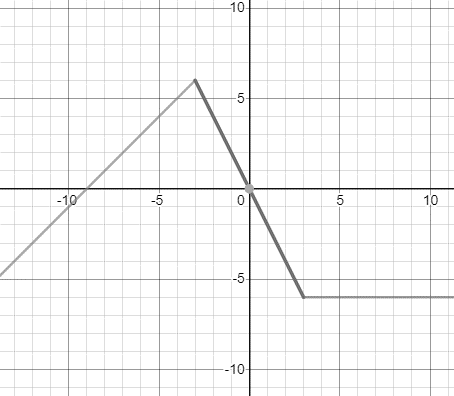# The domain and range of the function and sketch the graph.### Single Variable Calculus: Concepts...

4th Edition
James Stewart
Publisher: Cengage Learning
ISBN: 9781337687805### Single Variable Calculus: Concepts...

4th Edition
James Stewart
Publisher: Cengage Learning
ISBN: 9781337687805

#### Solutions

Chapter 1.1, Problem 46E
To determine

## To find: The domain and range of the function and sketch the graph.

Expert Solution

The given function is all real numbers and the is f(x)6 .

### Explanation of Solution

Given information:

The given function is,

f(x)={x+9ifx<32xif|x|36ifx>3

The given function is,

f(x)={x+9ifx<32xif|x|36ifx>3

The domain of the given function is all real numbersand the range of the function is f(x)6 .

Consider the graph of the given function,### Have a homework question?

Subscribe to bartleby learn! Ask subject matter experts 30 homework questions each month. Plus, you’ll have access to millions of step-by-step textbook answers!